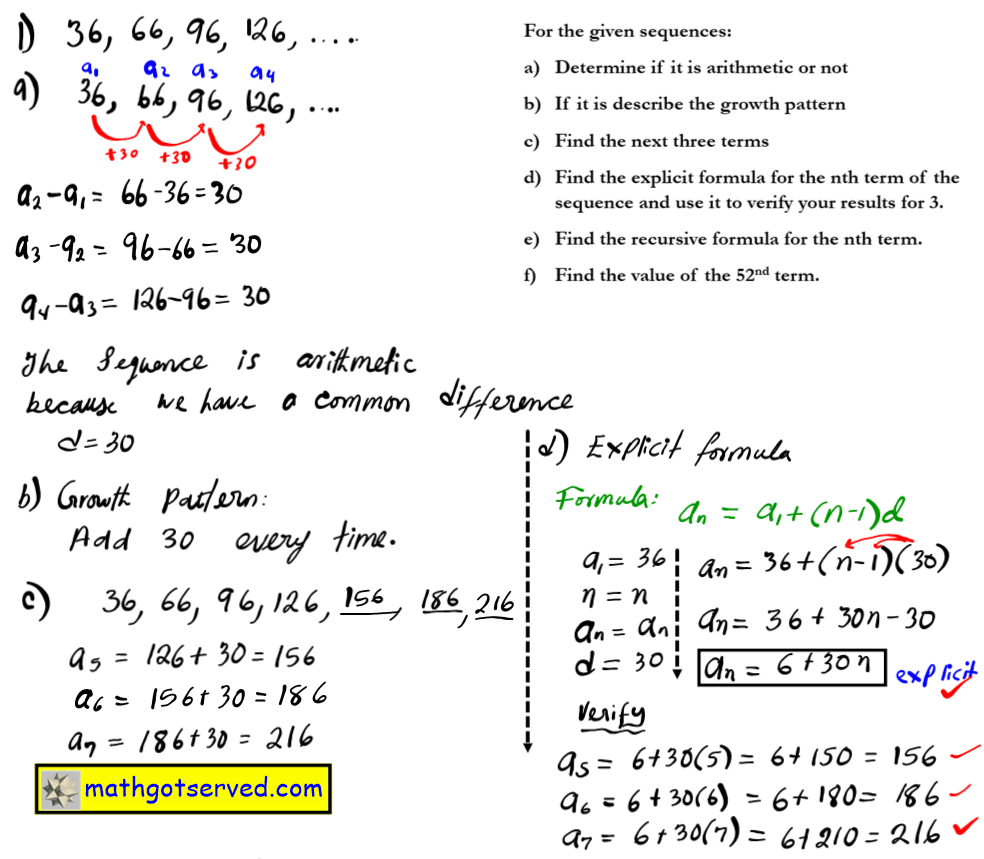MATHGOTSERVED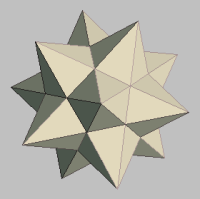Arithmetic & Geometric Sequences and Series
Definitions:
An Arithmetic Sequence is a list of terms each one differing from the previous by the same constant called the common difference.
Example:
10,7,4,1,-2
the common difference here is d=-3.​

Definitions:
An Arithmetic Sequence is a list of terms each one differing from the previous by the same constant called the common difference.
Example:
10,7,4,1,-2
the common difference here is d=-3.

An Arithmetic Series can be defined as the sum of an arithmetic sequence.
Example:
10+7+4+1+(-2)

Note: In an arithmetic sequence each term is seperated by commas. Where as in the case of an arithmetic series, each term is seperated from the next by pluses. A sequence is a list of numbers, and a series is the sum of nmbers.

Arithmetic Series Formula
The sum of the first n terms of an arithmetic series where a1 is the first term, and an is the nth term is given by the formula: (n/2)(a1+an)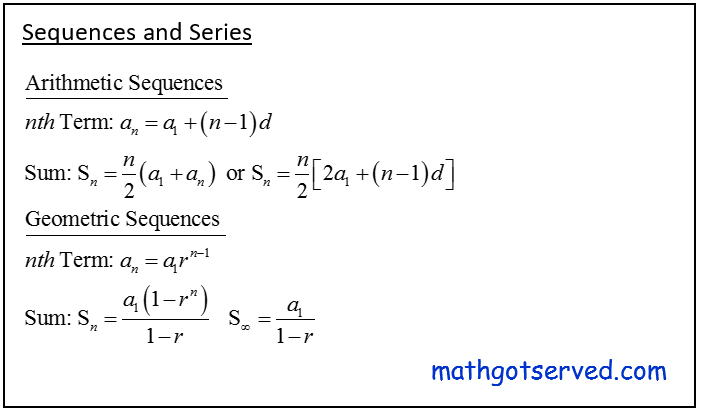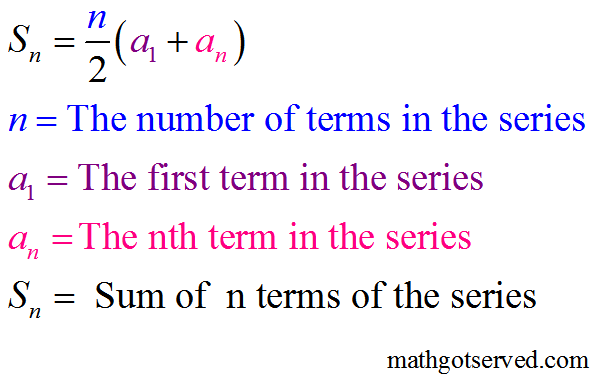An Arithmetic Series can be defined as the sum of an arithmetic sequence.
Example:
10+7+4+1+(-2)

Note: In an arithmetic sequence each term is seperated by commas. Where as in the case of an arithmetic series, each term is seperated from the next by pluses. A sequence is a list of numbers, and a series is the sum of nmbers.

Arithmetic Series Formula
The sum of the first n terms of an arithmetic series where a1 is the first term, and an is the nth term is given by the formula: (n/2)(a1+an)

PRACTICE TEST
SOLUTIONS TO  THE PRACTICE TEST
1.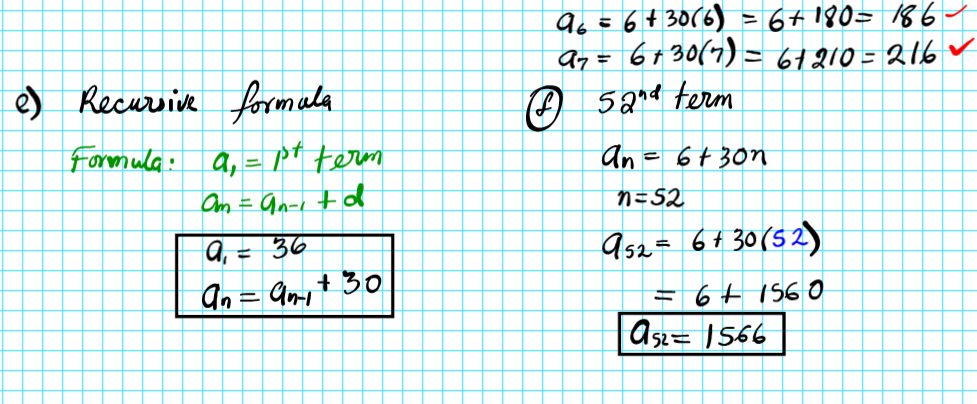2.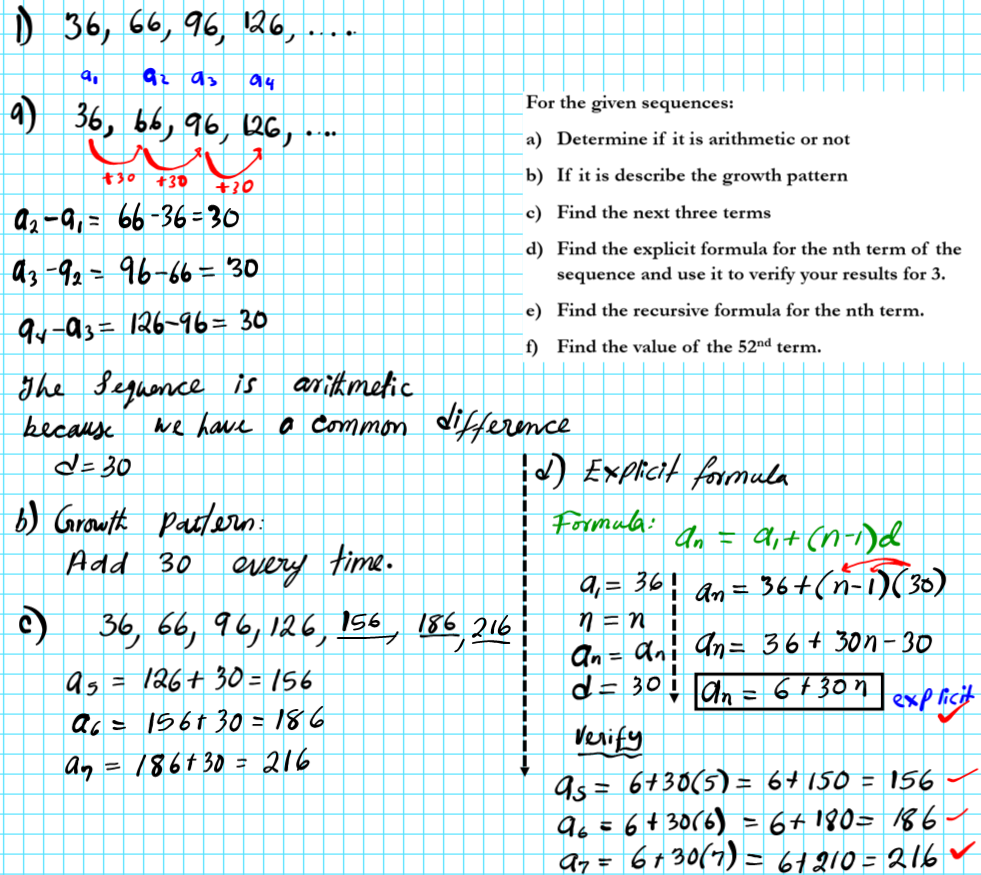3.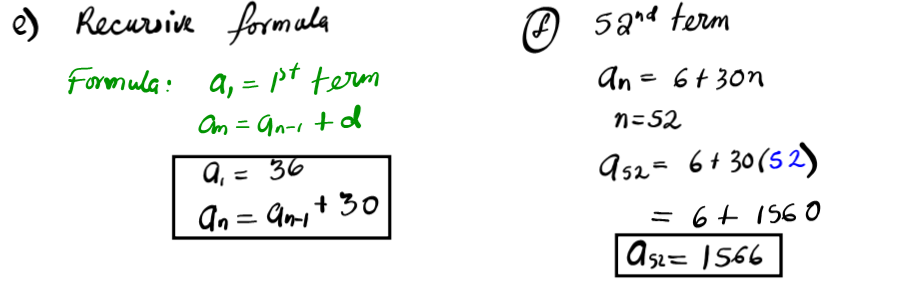4.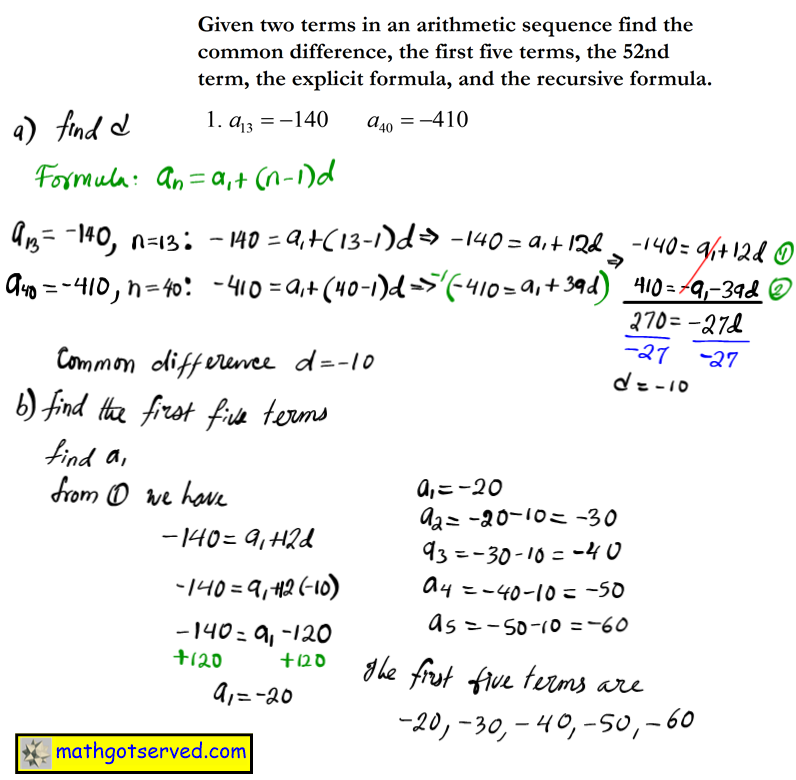5.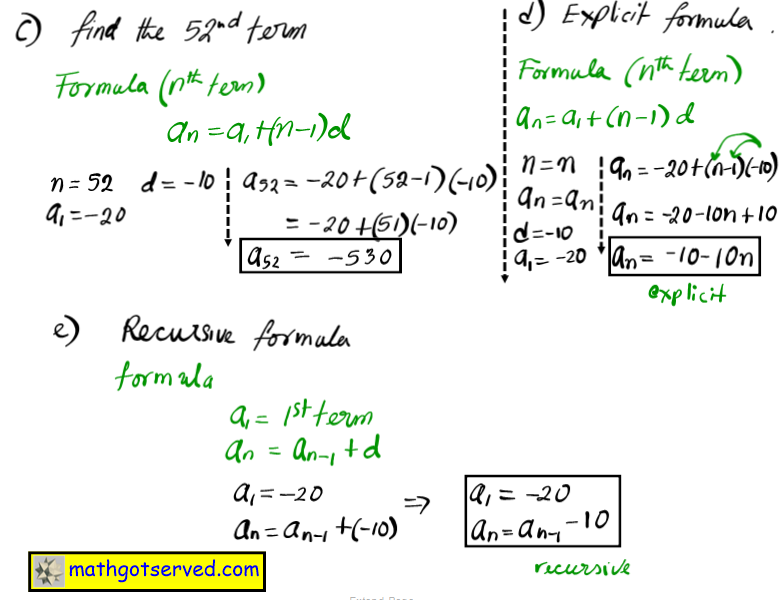6.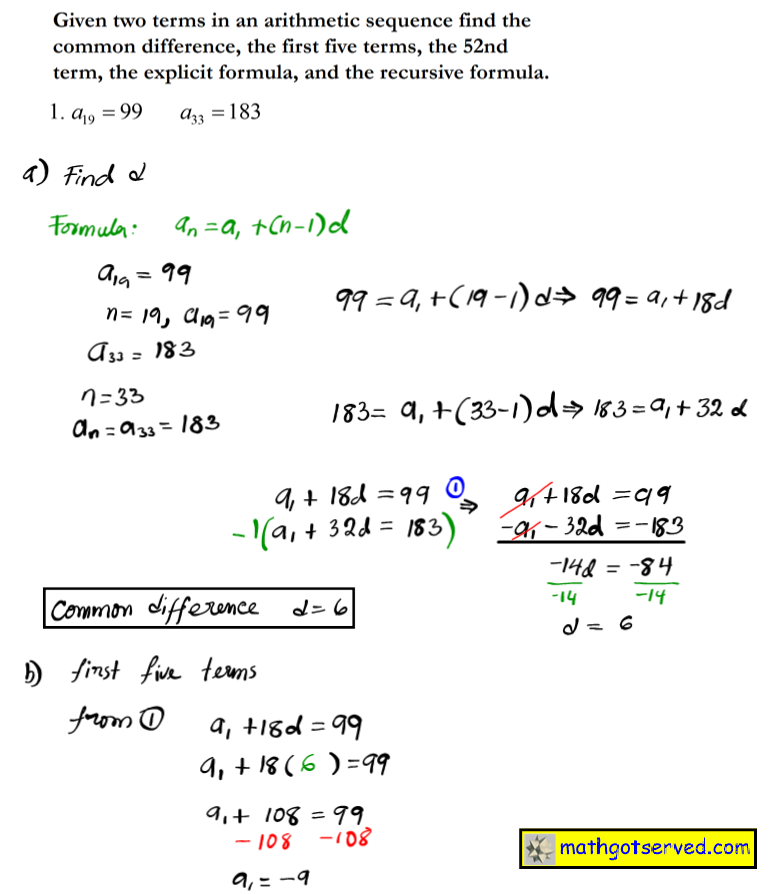7.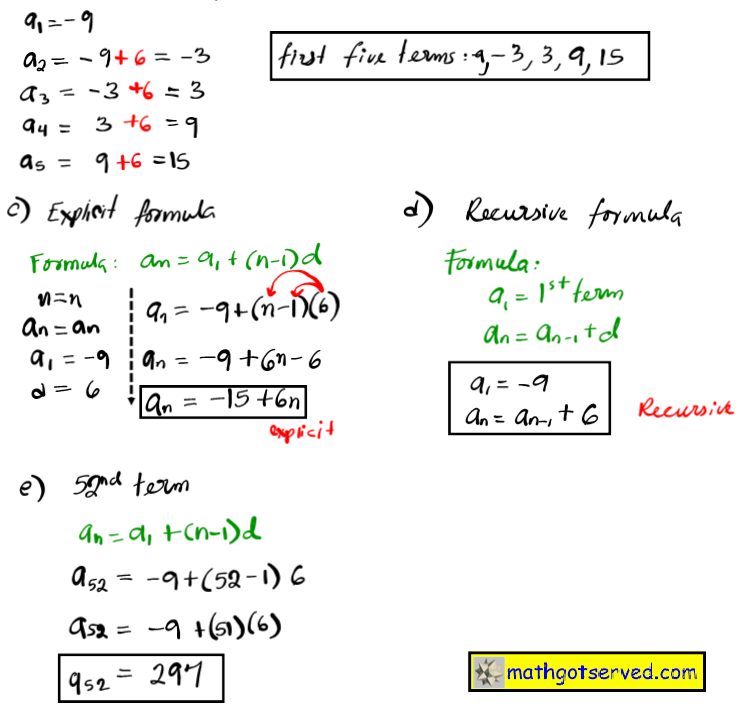8.